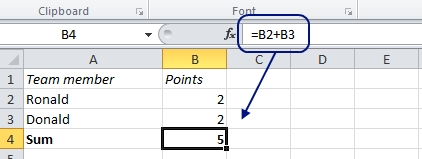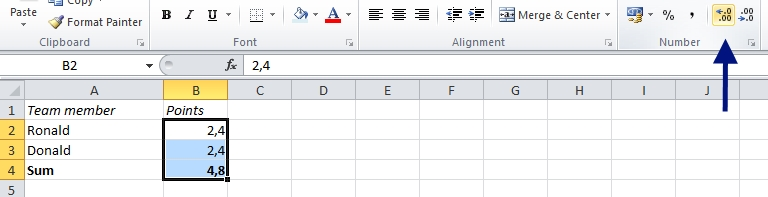# How to handle parts per million, basis points and per mille in Excel

Excel has built-in functionality to handle percent (%), but there is no automatic way to calculate parts per million, basis points, permyriad or per mille in Excel. It is easy to calculate though, but let’s start with the definitions:

And here’s how to apply it:

# Excel: 2 + 2 = 5

Can 2 +2 be 5?

Yes, at least if you look at this example in Excel:Of course, Excel doesn’t make mistakes like that, so there must be an explanation. Let’s try to increase the number of decimals:As it turns out, the real calculation wasn’t 2 + 2; it just looked like that. It was actually 2,4 + 2,4 = 4,8, but when you decrease the number of shown decimals to none, Excel displays the nearest integer in the cell, and it looks like 2 + 2 = 5. The actual number behind doesn’t change.

More Excel oddities:

Are you using a non-English version of Excel? Click here for translations of the 100 most common functions.